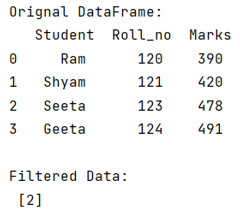# How to return the index of filtered values in pandas DataFrame?

Given a Pandas DataFrame, we have to return the index of filtered values.
Submitted by Pranit Sharma, on June 23, 2022

Pandas is a special tool that allows us to perform complex manipulations of data effectively and efficiently. Inside pandas, we mostly deal with a dataset in the form of DataFrame. DataFrames are 2-dimensional data structures in pandas. DataFrames consist of rows, columns, and the data.

To return the index of filtered values in pandas DataFrame, we will first filter the column values by comparing them against a specific condition, then we will use the index() method to return the index of the filtered value. We can also store all the filtered values into a list by using the tolist() method.

To work with pandas, we need to import pandas package first, below is the syntax:

```import pandas as pd
```

Let us understand with the help of an example,

## Python code to return the index of filtered values in pandas DataFrame

```# Importing pandas package
import pandas as pd

# Creating a dictionary
d= {
'Student':['Ram','Shyam','Seeta','Geeta'],
'Roll_no':[120,121,123,124],
'Marks':[390,420,478,491]
}

# Create a DataFrame
df = pd.DataFrame(d)

# Display original DataFrame
print("Orignal DataFrame:\n",df,"\n")

# Filter values
result = df[df['Student'] == 'Seeta'].index.tolist()

# Display result
print("Filtered Data:\n",result)
```

Output:Languages: » C » C++ » C++ STL » Java » Data Structure » C#.Net » Android » Kotlin » SQL
Web Technologies: » PHP » Python » JavaScript » CSS » Ajax » Node.js » Web programming/HTML
Solved programs: » C » C++ » DS » Java » C#
Aptitude que. & ans.: » C » C++ » Java » DBMS
Interview que. & ans.: » C » Embedded C » Java » SEO » HR
CS Subjects: » CS Basics » O.S. » Networks » DBMS » Embedded Systems » Cloud Computing
» Machine learning » CS Organizations » Linux » DOS
More: » Articles » Puzzles » News/Updates